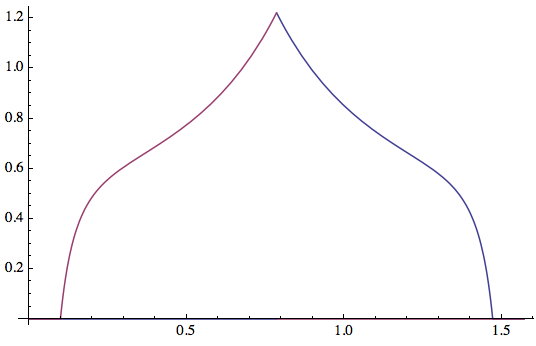# Random walk in a circle

Suppose we have a circle of radius $R$ centered in the origin of a $x,y$ cartesian reference frame. A particle starting from the center of the circle is moving with a speed given by: $$\overrightarrow{v}=\frac{1}{\sqrt{k^2+h^2}}v_0(k\underline i+h\underline j)$$ where $v_0=const$, $\underline i$ and $\underline j$ are the unit vectors of $x$ and $y$ and $k\in\mathbb{N},h\in\mathbb{N}$ random integer numbers uniformly distribuited and uncorrelated between them, such that $-\alpha\le k\le \alpha$, $-\alpha\le h\le \alpha$, $k\neq 0,h\neq 0$ and $\alpha\in\mathbb{N}$. Every time step $\Delta t$, a random $k$ and a random $h$ are picked and so the particle runs through a space $s=|\overrightarrow v|\Delta t$. The question is: calling $P(t)$ the probability to find the particle at the point $(x(t),y(t))$ at time $t$, after how many time steps the probability to find the particle outside the circle $(x(t)^2+y(t)^2)\gt R$ is: $P(t)\ge P_0?$ Thanks in advance.

• I'm not sure that I completely understand your question, but this sounds like effectively a one dimensional random walk where you're flipping a coin to decide whether you move closer to the starting point or further from it every step, so the distribution of distances your particle has from the starting point at large $t$ ought to be normal. – Aaron Golden Oct 2 '13 at 20:11
• I would say it's an anisotropic two-dimensional random walk, with fixed step size but random angle $\phi=\arctan (h/k)$ and $h,k$ uniformly distributed integers; for $\alpha\rightarrow\infty$, when the discreteness of the integers $h,k$ can be neglected, a simple closed form expression for $P(t)$ follows, but I would think the discreteness of $h,k$ for small $\alpha$ prevents a closed-form expression. – Carlo Beenakker Oct 2 '13 at 21:12
• @CarloBeenakker: suppose I don't have the discreteness of $h$ and $k$. Is it possible to find a solution in a closed form? – Riccardo.Alestra Oct 3 '13 at 8:50
• I'm sorry, I was overoptimistic --- no simple answer except for the diffusion approximation, when $P(x,y,t)\propto\exp[-(x^2+y^2)(v_0^2 t\Delta t)^{-1}]$. – Carlo Beenakker Oct 3 '13 at 13:32

## 1 Answer

This is mainly for reference, I have not found a simple answer outside of the diffusion approximation.

Assume $$\alpha\gg 1$$. The random walk has unit step size and the angle $$\phi$$ of a step with the $$x$$-axis is given by $$\phi=\arctan(h/k)$$ with $$h,k$$ two independent real numbers that are uniformly distributed in the range $$(1,\alpha)\cup(-1,-α)$$. The corresponding probability distribution $$P(\phi)$$ in the first quadrant $$(0<\phi<\pi/2)$$ is given by

$$P(\phi)=\frac{1}{2(\alpha-1)^2}\times\begin{cases}\alpha^2/\sin^2\phi-1/\cos^2\phi&{\rm if}\;\;1<\tan\phi<\alpha\\ \alpha^2/\cos^2\phi-1/\sin^2\phi&{\rm if}\;\;1/\alpha<\tan\phi<1\end{cases}$$

Here is a plot of $$P(\phi)$$ versus $$\phi$$ in the first quadrant for $$\alpha=10$$. (It is repeated identically in the other three quadrants.)As expected the steps have the largest probability at a 45 degree angle with the $$x$$ and $$y$$ axes.

So to find the probability distribution $$P(x,y,t)$$ after $$N=t/\Delta t$$ steps we seek the probability distribution of the complex number $$z=x+iy$$ given by

$$z=\sum_{n=1}^{N}\exp(i\phi_n)$$ with $$\phi_n$$, $$n=1,2,\ldots N$$ drawn independently from the distribution $$P(\phi)$$. Even for an isotropic distribution of $$P(\phi)=$$ constant, this has no simple answer (except for the large time limit, when the diffusion approximation holds and $$P(x,y,t)$$ is just a Gaussian).